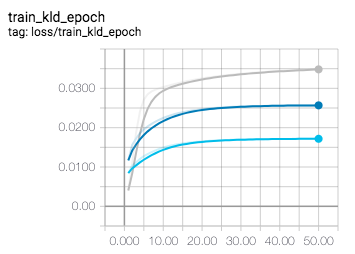# VAE example reparametrize

Hi everyone,

in the reparametrize function of the vae example, line 54 on github

    def reparameterize(self, mu, logvar):
std = torch.exp(0.5*logvar)
eps = torch.randn_like(std)


What is the theoretical reason to multiply the log variance vector with 0.5? What if you change it to 1 or 0.1?

Thanks niclas

1 Like

What if you change it to 1 or 0.1?

Good question. Then your data wouldn’t have unit variance (prob. doesn’t matter much in practice, you can maybe think of the spread of the distribution as a tuning param)

the logvar variable, which is the variance vector $\sigma^2$ of the covariance matrix $\Sigma = \sigma^2 * I$ is multiplied by 0,5.

What is the theoretical reason to do that?

Main reason is we work with the log for stability reasons. Hence, you have have

logVariance = log($\sigma^2$) = 2 * log(sigma)

To get the log standard deviation, you then basically divide by two

logStdDev = 2 * log(sigma) / 2 = 0.5 * 2 * log(sigma) = log(sigma)

4 Likes

That was quick, thanks a lot! I have trained different models on musical (MIDI) sequences and you are right in practice it does not really change a lot.

One thing i noticed though is that the KL divergence is lower if the parameter, lets call it c for now, is higher. So the grey plot used c=0.2 for this value the blue plot c=0.5 and for the turquoise plot i set it to c=1. Reconstruction loss is pretty much the same.Interesting! Maybe the intuition is sth along the lines of when you disentangle KL divergence, you can write it as cross-entropy - entropy, if you divide by a smaller c, the standard dev will be higher and your data will be more spread, and the entropy will be higher. Hence, the KL divergence will be lower by some value proportional to c.